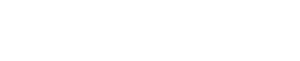# How to Calculate a z-score

Determining if something is probable in statistics can be tricky. I mean, how am I supposed to know if one measurement of a sample is particularly significant when compared to the entire sample or even the population? Well, luckily for us, statisticians of the highest caliber have calculated probabilities for us and we can calculate something called a z-score to determine significance.

## How the z-score came about

For a moment, let’s imagine that you have opened 118 bags of M&Ms and counted the total number of candies in each bag (thank you, by the way). When you take such a large number of measurements, one quick way to analyze the data is to calculate the mean, median, and mode of the data.

Another way to analyze the data is to compare the frequency or the number of occurrences for each value. Determining the frequency of each value allows you to build a histogram to look for overall trends in the data. The histogram for your M&M data is below.Do you notice that there is only one large hill in the data? That means that the data show a normal distribution. The normal distribution represents the tendency of that particular measurement to occur. In our data, there is a high chance that any random bag of M&Ms will contain 20 pieces of candy, whereas there is a very small chance of a random bag containing 23 candies.

A normal curve, shown below, is the statistical model of the distribution. It tells us how “normal” the data is.## How to calculate a z-score?

A z-score represents probability of a particular measure occurring. In our example, it would represent the probability of selecting a bag of M&Ms that contains a specific number of M&Ms. The z-score is calculated using the difference between an individual sample measure and in terms of the standard deviation.With the measure now in terms of standard deviations, we can use the normal curve. Calculating the z-score means you can determine the probability of a specific measure occurring. For example, you want to know how likely it is to get a package of M&Ms with 24 candies in it (enough to share, lol). So we calculate the z-score.Now that you have a z-score, what does it mean? Well, what you need to determine how likely that z-score is to occur. To do that, we have a nifty little chart that has calculated the probability of each z-score occurring.On one side is the value of the z-score. Across the top is the percentage of error that we are willing to accept and reject the null hypothesis. Notice that the value where the boxes meet is 0.9997. This represents the percentage of probabilities that are less than our z-score. It represents the probability of the null hypothesis occurring (e.g., the bag contains less than 24 candies.

In the case of the M&Ms, this means that the probability of getting a bag of M&Ms with less than 24 candies is 0.9997 or 99.97%. Which means there is at most 0.05 probability or 5% chance of getting a bag with 24 or more candies.

The z-score is essential to determining the probability of a measure occurring, which is also essential to hypothesis testing. Good thing you can calculate one now.